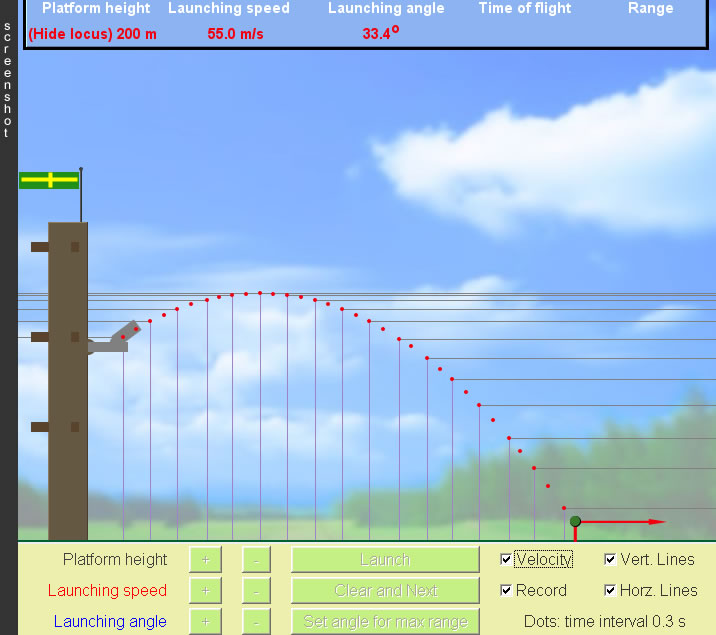Projectile Motion

* g = 10 N /kg

 Internal Links : 1D Kinematics Uniformly Accelerated Motion (Html5) Motion Applying force on object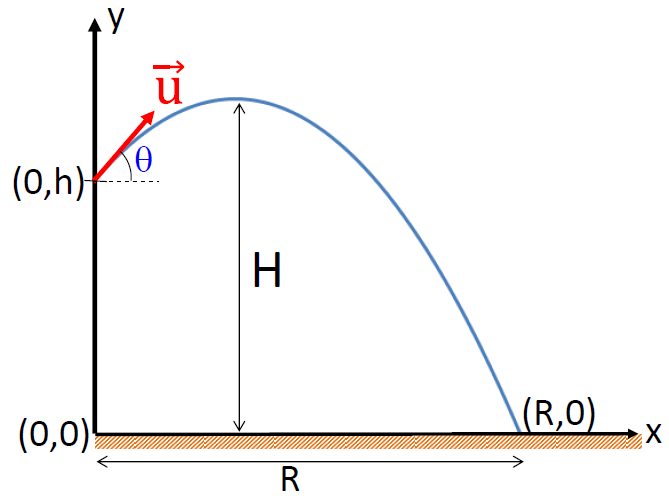Horizontal Motion Vertical Motion Initial Velocity ux = ucosθ uy = usinθ Acceleration ax = 0 ay = -g Velocity vx = ucosθ vy = usinθ - gt Position x = (ucosθ)t y = h + (usinθ)t - gt2/2
 Horizontal motion : uniform morion (constnat velocity) Vertical motion: uniformly accelerated motion When reaching the highest point, vy = 0 Maximum Height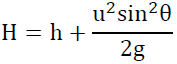When falling back to the ground, y = 0 Time of flight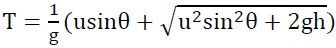Range R = (ucosθ)T Range is maximum when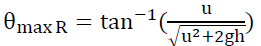In particular, when h = 0, θmax R = 45o

 The author (Chiu-king Ng) has the copyright on all the simulations in this website. Email phyAA@phy.hk, where AA is the prime number following 7. Last Update:2019-3-20